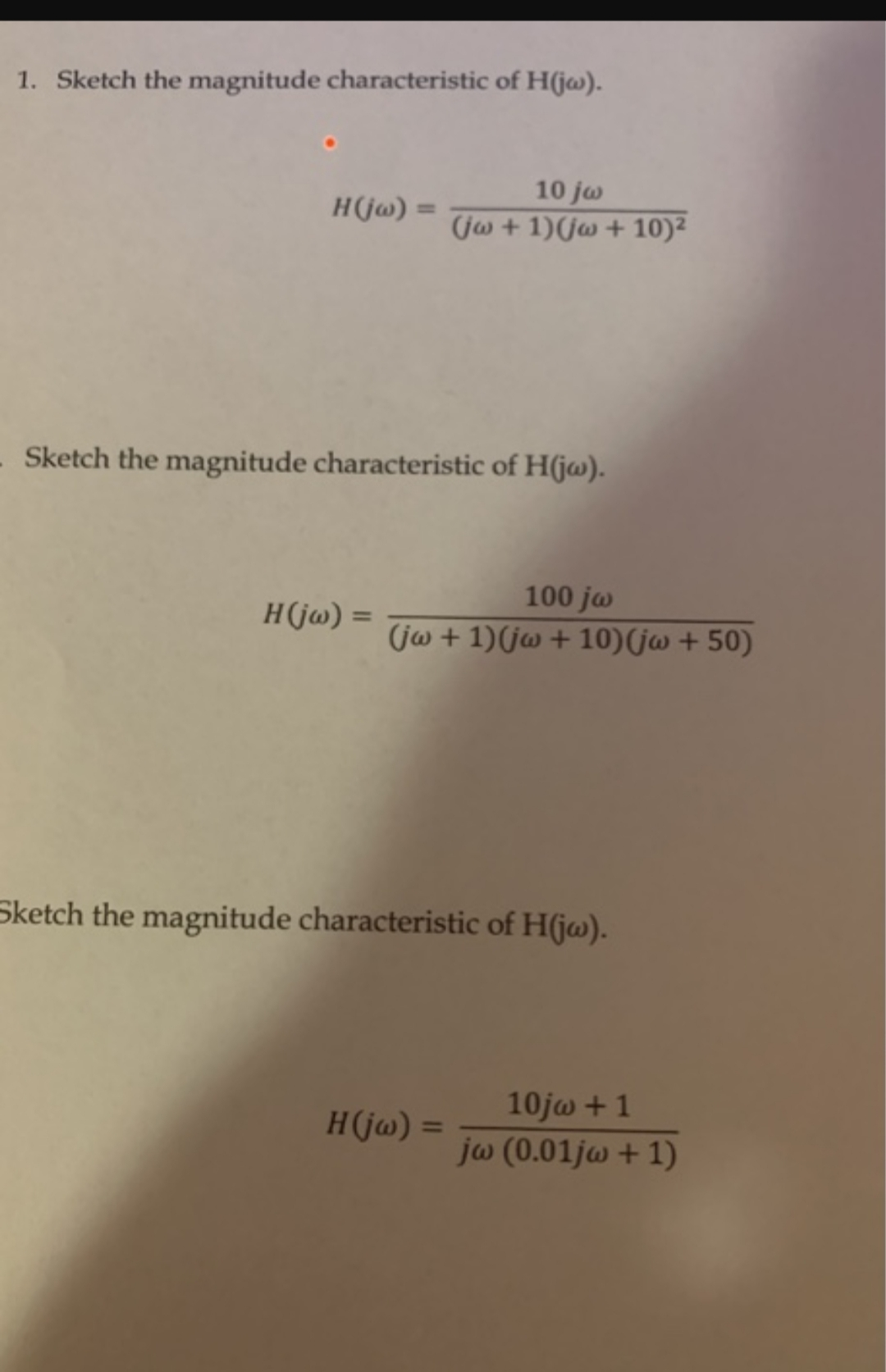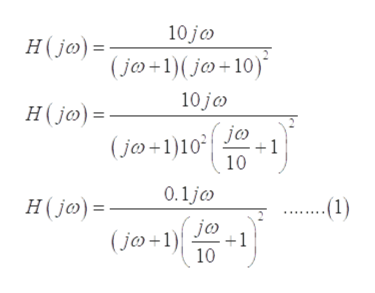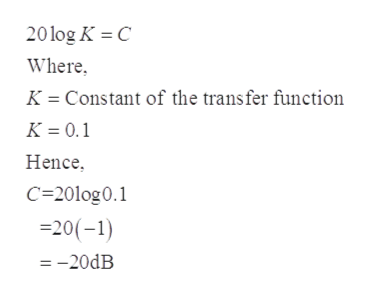# 1. Sketch the magnitude characteristic of H(jw).10 joH(ja)(ja+1)(j + 10)Sketch the magnitude characteristic of H(ja).100 jaH(ja) =(ja +1)j10) (ja +50)Sketch the magnitude characteristic of H(ja).10ja+1H(ja)1jw (0.01j+1)

Question
8 viewshelp_outlineImage Transcriptionclose1. Sketch the magnitude characteristic of H(jw). 10 jo H(ja) (ja+1)(j + 10) Sketch the magnitude characteristic of H(ja). 100 ja H(ja) = (ja +1)j10) (ja +50) Sketch the magnitude characteristic of H(ja). 10ja+1 H(ja) 1 jw (0.01j+1) fullscreen
check_circle

Step 1

We are authorized to answer one question at a time, since you have not mentioned which question you are looking for, so we are answering the first one, please repost your other question separately if you want to get answered.

Step 2

For sketching the magnitude characteristics graph for the given transfer function, firstly write it in the pole zero form to calculate the pole and zero of the given function:help_outlineImage Transcriptionclose10 jo H(jo) (jo+1(jo+10) 10 jc H(jo) jo 1 (jo+1)10 10 0.1j ...1) H(jo) = jc (jo+1 +1 10 fullscreen
Step 3

From the above equation, the position of poles and zeroes.

Three poles are at 1, 10, 10 and one zero is at 0.

Where, the zeroes contribute in positive 20-De...help_outlineImage Transcriptionclose20log K C Where Constant of the transfer function K K 0.1 Hence C 20log0.1 20(-1) =-20dB fullscreen

### Want to see the full answer?

See Solution

#### Want to see this answer and more?

Solutions are written by subject experts who are available 24/7. Questions are typically answered within 1 hour.*

See Solution
*Response times may vary by subject and question.
Tagged in

### Electrical Engineering